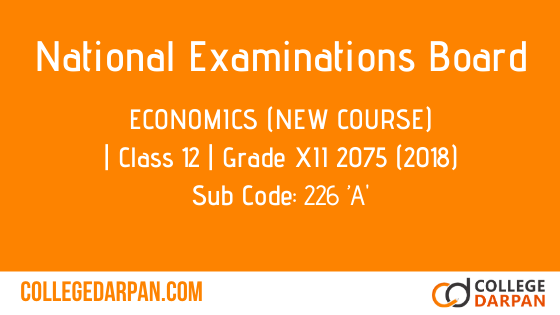# NEB- Grade XII 2075 (2018) Economics (New Course)(226 ’A)

Subject Code: 226 ’A’

2075 (2018)

Economics

(New Course)

Candidates are required to give their answers in their own words as far as practicable. The figures in the margin indicate full marks.

Time – 3 hrs

Full Marks – 100

(Part-1)

(Group ‘A’)

(Attempt any Two questions) [2×10=20]

1. (Explain the law of diminishing marginal utility. What are its exceptions?) [7+3]

2. (Explain the derivation of short-run total cost curves.)

3.(Explain the Ricardian theory of rent with criticism.) [7+3]

(Group ‘B’)

(Attempt any    four questions) [4×5=20]

4. (Define price elasticity of demand with its types.)

5. (Explain the law of increasing returns to scale.)

6. (Explain the derivation of AR and MR from TR under monopoly market.)

7. (How are price and output determined under perfect competition?)

8. (Explain the wage fund theory of wage.)

(Group ‘C’)

(Attempt  all questions) [5×2=10]

9. (What is income elasticity of demand?)
10. (What is consumer’s surplus?)
11. (What is production?)
12. (Write any four features of perfect competitive market.)
13. (What is meant by firm equilibrium?)

(Part-2)

(Group ‘A’)

(Attempt any    Two questions) [2×10=20]

14. (What is central bank? Explain its functions.) [2+8]

15. (Define direct tax with its merits and demerits.) [2+4+4]

16. (Explain the comparative cost theory of international trade.) 

(Group ‘B’)

(Attempt any    four questions) [4×5=20]

17. (Define national income with different concepts.)

18. (Explain the quantity theory of money.)

19. (Explain the functions of commercial bank.)

20. (Explain the process of budget formulation in Nepal.)

(Group ‘C’)